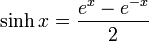# Hyperbolic sine function

(Redirected from Sinh)
The hyperbolic sine function, denoted$\sinh$, is defined as follows:$\sinh x = \frac{e^x - e^{-x}}{2}$
Here,$e^x$ denotes the exponential function.